# Bookshop management system using file handling

• Difficulty Level : Medium
• Last Updated : 04 Feb, 2021

The task is to build the bookshop management system using file handling in C++ that helps to keep track of all the book records in a file. Below are the features to be implemented:

• Add new book and the details of the book are:
• Book ID
• Name of book
• Name of Author
• Number of books
• Delete a book
• Update a book
• View all the books
• Exit

Approach:

Want to learn from the best curated videos and practice problems, check out the C++ Foundation Course for Basic to Advanced C++ and C++ STL Course for the language and STL. To complete your preparation from learning a language to DS Algo and many more, please refer Complete Interview Preparation Course.
• All the functions will be provided under switch cases.
• The concept of file handling will be used to write the data in a text file and read the written data as well.
• The required function will be written under the switch cases which will be executed as per the input of the user.
• Simple file handing concepts like opening a file, closing a file, writing in a file and reading the file, etc. are used to develop the code.
• An IDE for compiling C++ language code.

Below is the implementation of the above approach:

## C++

 `// C++ program to illustrate bookshop``// management system using File Handling``#include ``#include ``#include ``#include ``using` `namespace` `std;`` ` `// Bookshop Class``class` `bookshope {``public``:``    ``void` `control_panel();``    ``void` `add_book();``    ``void` `show_book();``    ``void` `check_book();``    ``void` `update_book();``    ``void` `del_book();``};`` ` `// Function to display the menus``void` `bookshope::control_panel()``{``    ``system``(``"cls"``);``    ``cout << ``"\n\n\t\t\t\tCONTROL  PANEL"``;``    ``cout << ``"\n\n1. ADD BOOK"``;``    ``cout << ``"\n2. DISPLAY BOOKS"``;``    ``cout << ``"\n3. CHECK PARTICULAR BOOK"``;``    ``cout << ``"\n4. UPDATE BOOK"``;``    ``cout << ``"\n5. DELETE BOOK"``;``    ``cout << ``"\n6. EXIT"``;``}`` ` `// Function to add book``void` `bookshope::add_book()``{``    ``system``(``"cls"``);``    ``fstream file;``    ``int` `no_copy;``    ``string b_name, a_name, b_id;``    ``cout << ``"\n\n\t\t\t\tADD BOOKS"``;``    ``cout << ``"\n\nBook ID : "``;``    ``cin >> b_id;``    ``cout << ``"\nBook Name : "``;``    ``cin >> b_name;``    ``cout << ``"\nAuthor Name : "``;``    ``cin >> a_name;``    ``cout << ``"\nNo. of Books : "``;``    ``cin >> no_copy;`` ` `    ``// Open file in append or``    ``// output mode``    ``file.open(``"D:// book.txt"``,``              ``ios::out | ios::app);``    ``file << ``" "` `<< b_id << ``" "``         ``<< b_name << ``" "` `<< a_name``         ``<< ``" "` `<< no_copy << ``"\n"``;``    ``file.close();``}`` ` `// Function to display book``void` `bookshope::show_book()``{``    ``system``(``"cls"``);``    ``fstream file;``    ``int` `no_copy;``    ``string b_name, b_id, a_name;``    ``cout << ``"\n\n\t\t\t\t\tAll BOOKS"``;`` ` `    ``// Open the file in input mode``    ``file.open(``"D:// book.txt"``, ios::in);``    ``if` `(!file)``        ``cout << ``"\n\nFile Opening Error!"``;``    ``else` `{`` ` `        ``cout << ``"\n\n\nBook ID\t\tBook"``             ``<< ``"\t\tAuthor\t\tNo. of "``                ``"Books\n\n"``;``        ``file >> b_id >> b_name;``        ``file >> a_name >> no_copy;`` ` `        ``// Till end of file is reached``        ``while` `(!file.eof()) {`` ` `            ``cout << ``"  "` `<< b_id``                 ``<< ``"\t\t"` `<< b_name``                 ``<< ``"\t\t"` `<< a_name``                 ``<< ``"\t\t"` `<< no_copy``                 ``<< ``"\n\n"``;``            ``file >> b_id >> b_name;``            ``file >> a_name >> no_copy;``        ``}`` ` `        ``system``(``"pause"``);`` ` `        ``// Close the file``        ``file.close();``    ``}``}`` ` `// Function to check the book``void` `bookshope::check_book()``{``    ``system``(``"cls"``);``    ``fstream file;``    ``int` `no_copy, count = 0;``    ``string b_id, b_name, a_name, b_idd;`` ` `    ``cout << ``"\n\n\t\t\t\tCheck "``         ``<< ``"Particular Book"``;`` ` `    ``// Open the file in input mode``    ``file.open(``"D:// book.txt"``, ios::in);``    ``if` `(!file)``        ``cout << ``"\n\nFile Opening Error!"``;``    ``else` `{`` ` `        ``cout << ``"\n\nBook ID : "``;``        ``cin >> b_idd;``        ``file >> b_id >> b_name;``        ``file >> a_name >> no_copy;`` ` `        ``while` `(!file.eof()) {`` ` `            ``if` `(b_idd == b_id) {`` ` `                ``system``(``"cls"``);``                ``cout << ``"\n\n\t\t\t\t"``                     ``<< ``"Check Particular Book"``;``                ``cout << ``"\n\nBook ID : "``                     ``<< b_id;``                ``cout << ``"\nName : "``                     ``<< b_name;``                ``cout << ``"\nAuthor : "``                     ``<< a_name;``                ``cout << ``"\nNo. of Books : "``                     ``<< no_copy;``                ``cout << endl``                     ``<< endl;``                ``count++;``                ``break``;``            ``}``            ``file >> b_id >> b_name;``            ``file >> a_name >> no_copy;``        ``}``        ``system``(``"pause"``);``        ``file.close();``        ``if` `(count == 0)``            ``cout << ``"\n\nBook ID Not"``                 ``<< ``" Found..."``;``    ``}``}`` ` `// Function to update the book``void` `bookshope::update_book()``{``    ``system``(``"cls"``);``    ``fstream file, file1;``    ``int` `no_copy, no_co, count = 0;``    ``string b_name, b_na, a_name;``    ``string a_na, b_idd, b_id;`` ` `    ``cout << ``"\n\n\t\t\t\tUpdate Book Record"``;``    ``file1.open(``"D:// book1.txt"``,``               ``ios::app | ios::out);``    ``file.open(``"D:// book.txt"``, ios::in);`` ` `    ``if` `(!file)``        ``cout << ``"\n\nFile Opening Error!"``;``    ``else` `{`` ` `        ``cout << ``"\n\nBook ID : "``;``        ``cin >> b_id;``        ``file >> b_idd >> b_name;``        ``file >> a_name >> no_copy;`` ` `        ``// Till end of file is reached``        ``while` `(!file.eof()) {``            ``if` `(b_id == b_idd) {``                ``system``(``"cls"``);``                ``cout << ``"\t\t\t\t"``                     ``<< ``"Update Book Record"``;``                ``cout << ``"\n\nNew Book Name : "``;``                ``cin >> b_na;``                ``cout << ``"\nAuthor Name : "``;``                ``cin >> a_na;``                ``cout << ``"\nNo. of Books : "``;``                ``cin >> no_co;``                ``file1 << ``" "` `<< b_id << ``" "``                      ``<< b_na << ``" "``                      ``<< a_na << ``" "` `<< no_co``                      ``<< ``"\n\n"``;``                ``count++;``            ``}``            ``else``                ``file1 << ``" "` `<< b_idd``                      ``<< ``" "` `<< b_name``                      ``<< ``" "` `<< a_name``                      ``<< ``" "` `<< no_copy``                      ``<< ``"\n\n"``;``            ``file >> b_idd >> b_name;``            ``file >> a_name >> no_copy;``        ``}``        ``if` `(count == 0)``            ``cout << ``"\n\nBook ID"``                 ``<< ``" Not Found..."``;``    ``}``    ``cout << endl;``    ``system``(``"pause"``);`` ` `    ``// Close the files``    ``file.close();``    ``file1.close();``    ``remove``(``"D:// book.txt"``);``    ``rename``(``"D:// book1.txt"``,``           ``"D:// book.txt"``);``}`` ` `// Function to delete book``void` `bookshope::del_book()``{``    ``system``(``"cls"``);``    ``fstream file, file1;``    ``int` `no_copy, count = 0;``    ``string b_id, b_idd, b_name, a_name;``    ``cout << ``"\n\n\t\t\t\tDelete a Book"``;`` ` `    ``// Append file in output mode``    ``file1.open(``"D:// book1.txt"``,``               ``ios::app | ios::out);``    ``file.open(``"D:// book.txt"``,``              ``ios::in);`` ` `    ``if` `(!file)``        ``cout << ``"\n\nFile Opening Error..."``;``    ``else` `{`` ` `        ``cout << ``"\n\nBook ID : "``;``        ``cin >> b_id;``        ``file >> b_idd >> b_name;``        ``file >> a_name >> no_copy;``        ``while` `(!file.eof()) {`` ` `            ``if` `(b_id == b_idd) {`` ` `                ``system``(``"cls"``);``                ``cout << ``"\n\n\t\t\t\t"``                     ``<< ``"Delete a Book"``;``                ``cout << ``"\n\nBook is Deleted "``                        ``"Successfully...\n\n"``;``                ``count++;``            ``}``            ``else``                ``file1 << ``" "` `<< b_idd``                      ``<< ``" "` `<< b_name``                      ``<< ``" "` `<< a_name``                      ``<< ``" "` `<< no_copy``                      ``<< ``"\n\n"``;``            ``file >> b_idd >> b_name;``            ``file >> a_name >> no_copy;``        ``}``        ``if` `(count == 0)``            ``cout << ``"\n\nBook ID "``                 ``<< ``"Not Found..."``;``    ``}``    ``system``(``"pause"``);`` ` `    ``// Close the file``    ``file.close();``    ``file1.close();``    ``remove``(``"D:// book.txt"``);``    ``rename``(``"D:// book1.txt"``,``           ``"D:// book.txt"``);``}`` ` `// Function for book shop record``void` `bookShopRecord()``{``    ``int` `choice;``    ``char` `x;``    ``bookshope b;`` ` `    ``while` `(1) {`` ` `        ``b.control_panel();``        ``cout << ``"\n\nEnter your choice : "``;``        ``cin >> choice;``        ``switch` `(choice) {`` ` `        ``case` `1:``            ``do` `{`` ` `                ``b.add_book();``                ``cout << ``"\n\nWant to add"``                     ``<< ``" another book? "``                        ``"(y/n) : "``;``                ``cin >> x;``            ``} ``while` `(x == ``'y'``);``            ``break``;`` ` `        ``case` `2:``            ``b.show_book();``            ``break``;`` ` `        ``case` `3:``            ``b.check_book();``            ``break``;``        ``case` `4:``            ``b.update_book();``            ``break``;`` ` `        ``case` `5:``            ``b.del_book();``            ``break``;`` ` `        ``case` `6:``            ``exit``(0);``            ``break``;`` ` `        ``default``:``            ``cout << ``"\n\nINVALID CHOICE\n"``;``        ``}``    ``}``}`` ` `// Driver Code``int` `main()``{``    ``// Function Call``    ``bookShopRecord();`` ` `    ``return` `0;``}`

Output: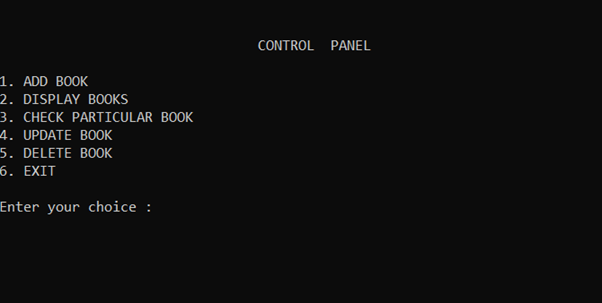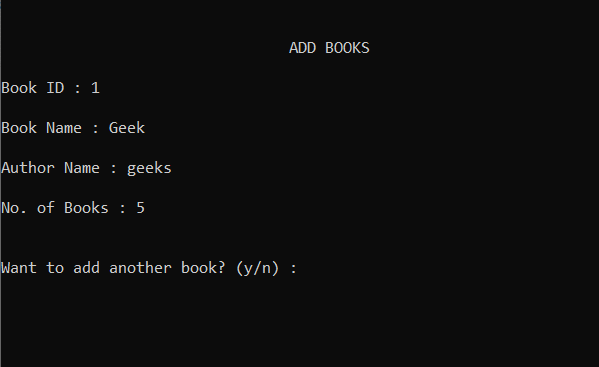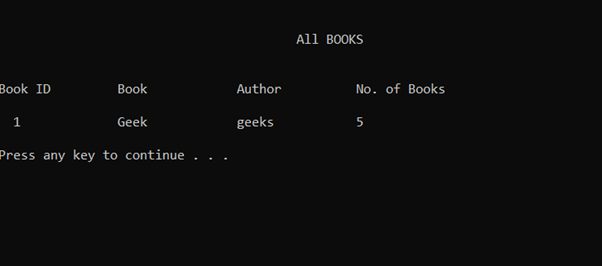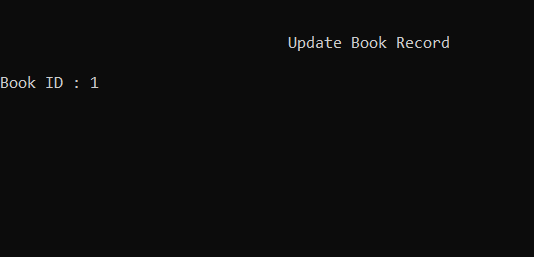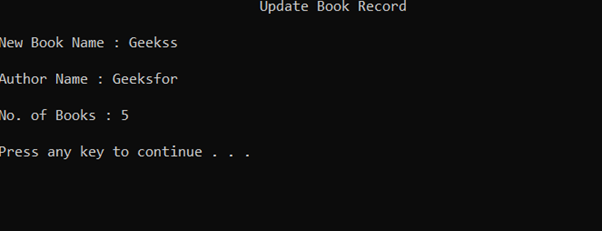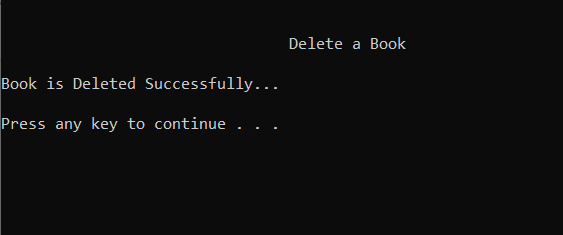My Personal Notes arrow_drop_up